# EMF Equation of a DC Generator

When the armature rotates in a magnetic field, a conductor of armature coil cut the magnetic flux and EMF get induced in it. The induced EMF in a coil depend upon the speed of armature and the flux produced by field winding.

In case of generator, the EMF induced in rotating armature called as generated EMF and it is denoted by Eg. But in case of motor EMF induced in rotating coil is known as Back EMF and it is denoted by Eb.

Now let’s start to derive the equation of EMF induced in DC Generator. Before starting the derivation you can learn about construction of DC generator.

## EMF Equation of DC Generator

Question: Derive the EMF Equation of DC generator.

When a mechanical device, such as a diesel engine or any other prime movers the drives armature with shaft, armature winding cuts the magnetic flux produced by the field winding on pole.

An equation for EMF induced can be obtained as under.

Let,

• P = Number of poles of the generator.
• Φ= Flux produced by each pole, Wb.
• N = Speed in R.P.M. at which the generator is driven.
• Z = Number of conductors of armature winding.
• A = Number of parallel paths of armature winding.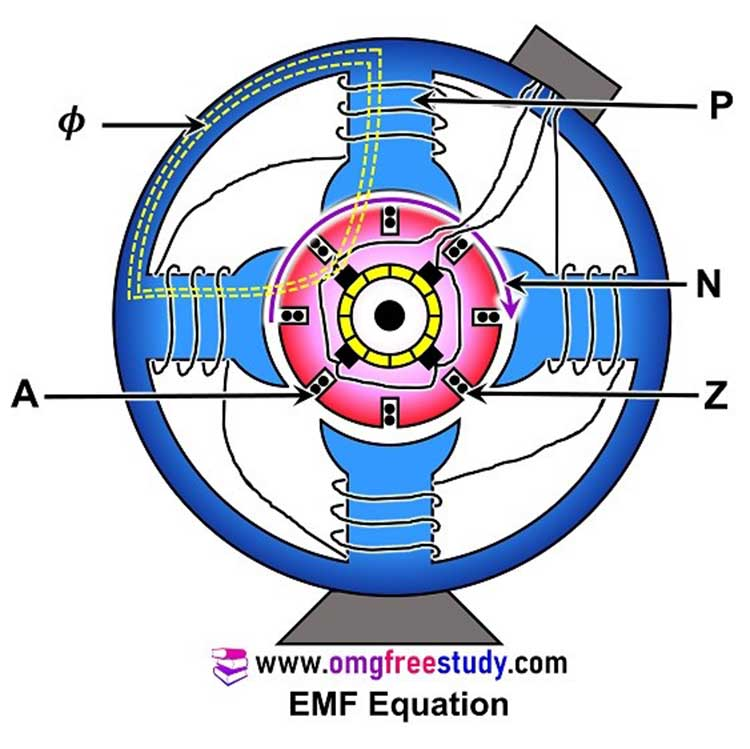Then according to Faraday’s law of induction, magnitude of E.M.F. induced in a conductor is,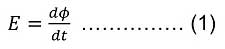For one complete revolution of a conductor, the flux cut by the conductor is (PΦ) Wb.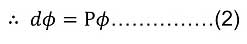And time required to complete one revolution is (60/N) seconds.  Therefore, Equation may be written as,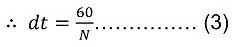Now, Put equation 2 & 3 in equation number (1). we get,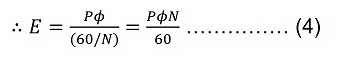As “Z” conductors are divided in “A” parallel groups, there are Z/A conductors in series in each group of winding.

Put this value in equation (4)

Therefore EMF induced (Eg) in the total armature winding is,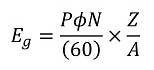This is the final EMF Equation of a DC Generator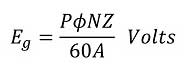The above equation will change with winding connection as follows:

In wave wound generator
Numbers of parallel paths (A)= 2
Therefore equation becomes,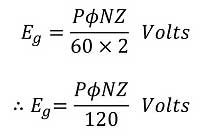In lap wound generator
Number of parallel paths (A) is equal to number of pole (P). i.e. A=P

Therefore equation becomes,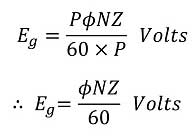So, You can Watch MCQ Question Lecture on topic DC Motor and DC Generator on YouTube by clicking here.

### 4 thoughts on “EMF Equation of a DC Generator”

1.2.